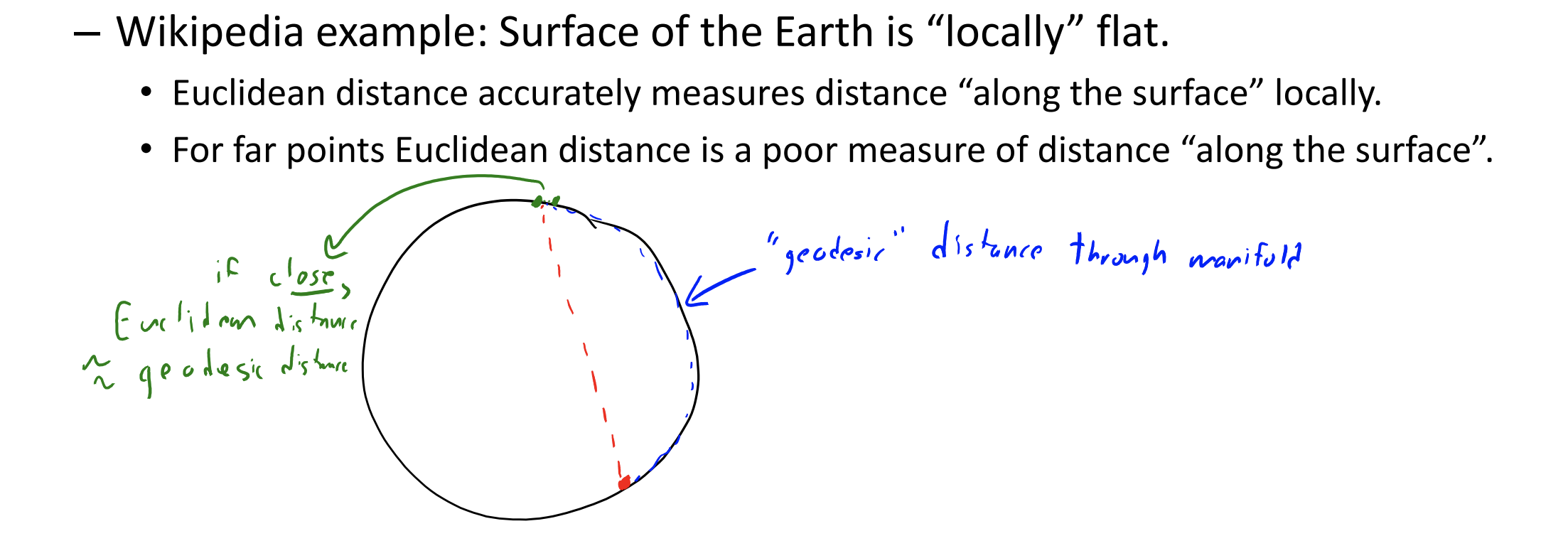Like change of basis but instead of hand-picking the features, we learn them from data.

Part weights are a change of basis from to some . The canonical example is Principal Component Analysis (PCA)

## PCA §

PCA is parametric and does not provide unique global optimum.

Takes in a matrix and an input and outputs two matrices such that :

• is a (n,k) matrix. Each row is a set of features/part weights
• W is a (k,d) matrix. Each row is a part/factor/principle component
• We can think of as rotating data so that the slope is zero
• Approximation of one is
• Each can be thought of as a linear combination of all the factors

Assumptions:

• Assumes is centered (each column of has a mean of zero)

Use cases:

• Dimension reductionality: Effectively allows us to reduce the dimensionality of X if
• Actually, it only ever makes sense if
• Outlier detection: if PCA gives a poor approximation, could be an outlier
• Data visualization: to visualize high-dimensional objects

We minimize

If we do alternating minimization,

1. Fix Z and optimize W:
2. Fix W and optimize Z:

We converge to a local optimum which will be a global optimum if W and Z are randomly initialized (if you don’t pick a saddle point)

For large X, we can also just use SGD and cost per iteration is only

### Choose by variance explained §

How much of the variance can be explained by the choice of factors?

For a given , we compute the variance of the errors over the variable of each given

### Uniqueness §

Optimal is non-unique:

1. Scaling problem: Can multiply any by any non-zero
2. Rotation problem: Can rotate any within the span
3. Label switching problem: Can switch any with any other

To help with uniqueness,

1. Normalization: We ensure
2. Orthogonality: We enforce for all
3. Sequential fitting, we fit each in sequence

## Multi-Dimensional Scaling §

Gradient descent on points on a scatter point; try to make scatterplot distances match high-dimensional distances

No matrix needed! However, cannot be done using singular value decomposition (a matrix factoring technique). We need gradient descent.

• Non convex
• Sensitive to initialization
• Unfortunately, MDS often does not work well in practice; MDS tends to “crowd/squash” all the data points together like PCA.

## t-SNE §

t-Distributed Stochastic Neighbour Embedding

However, using Euclidean (L2-norm) may not be a great representation of data that lives on low-dimensional manifolds. In these cases, Euclidean distances make sense “locally” but Euclidean distances may not make sense “globally”.t-SNE is actually a special case of Multi-Dimensional Scaling. The key idea is to focus on distance to “neighbours”, allowing gaps between distances to grow

## Word2Vec §

Each word is represented by a vector of real numbers